# Laboratory Goals

The objectives of this laboratory session are as follows:

• Building a 8-bit R2R DAC in LTSPICE with measured resistor values.

• Simulation of ramp test and measuring settling time,INL,DNL values.

• Simulation of sine measurement of R2R DAC with different signal frequencies.

• Measuring the INL,DNL and FFT with calibrated sine pattern.

# 8-bit R2R DAC.

The following figure 1 shows the real implementation of 8-bit R2R DAC on a breadboard.
Here we have the resistance of R and 2R. Input of DAC connected to digital pins, output connected to the digital oscilloscope.
Figure 2 shows LTSPICE schematic of R2R DAC with measured resistance values.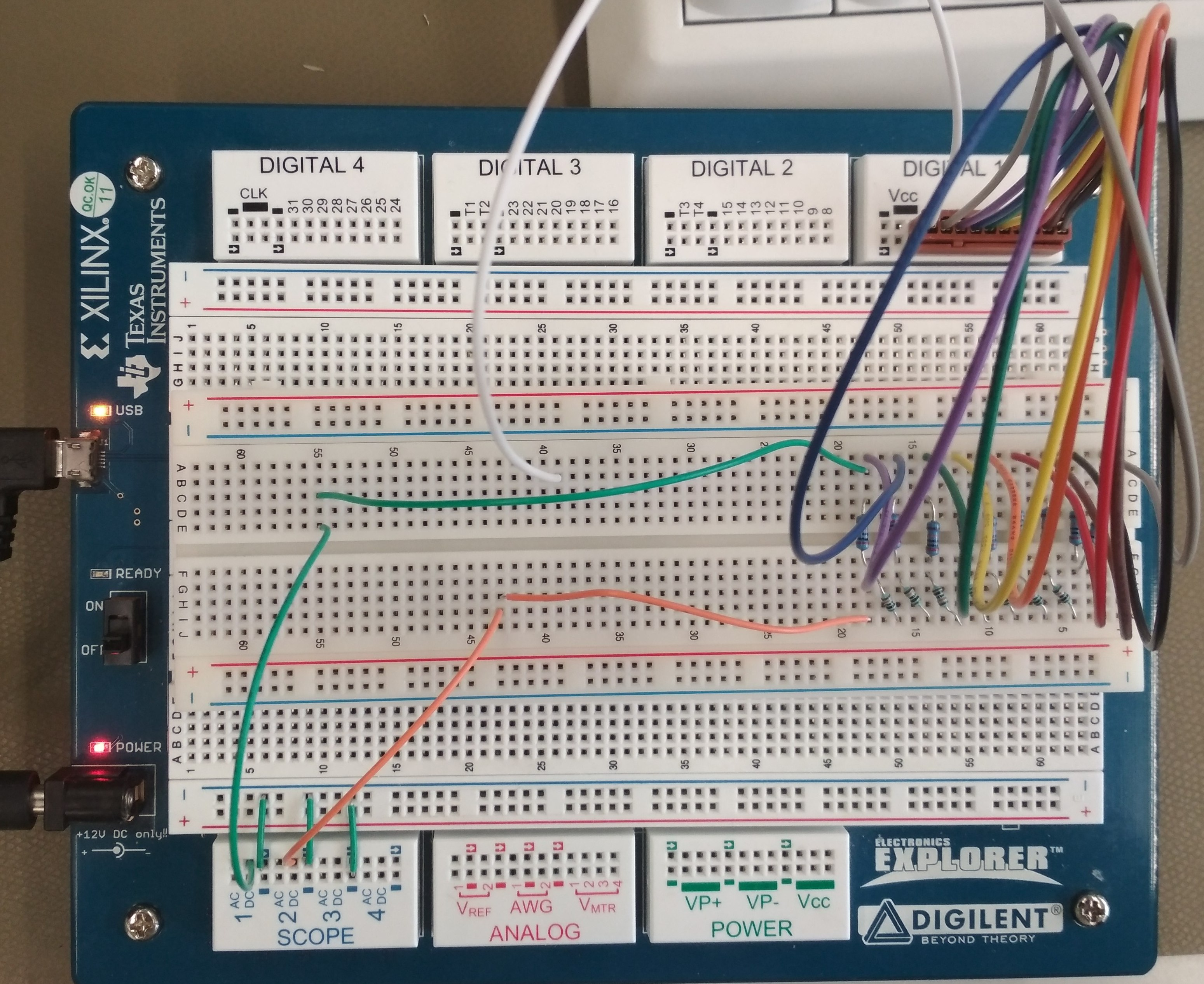figure 1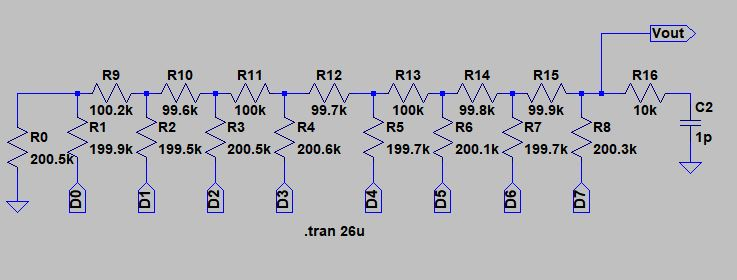figure 2

# LTSPICE schematic with resistor values.

 Resistor values (ohm) Resistor values (ohm) R0 200.5K R8 200.3K R1 199.9K R9 100.2K R2 199.5K R10 99.6K R3 200.5K R11 100K R4 200.6K R12 99.7K R5 199.7K R13 100K R6 200.1K R14 99.8K R7 199.7K R15 99.9K

Table 1

# Simulation of Ramp test

 The simulation of Ramp test for different frequencies are shown in the figure. The frequencies are varied from 10 Khz to 10 Mhz.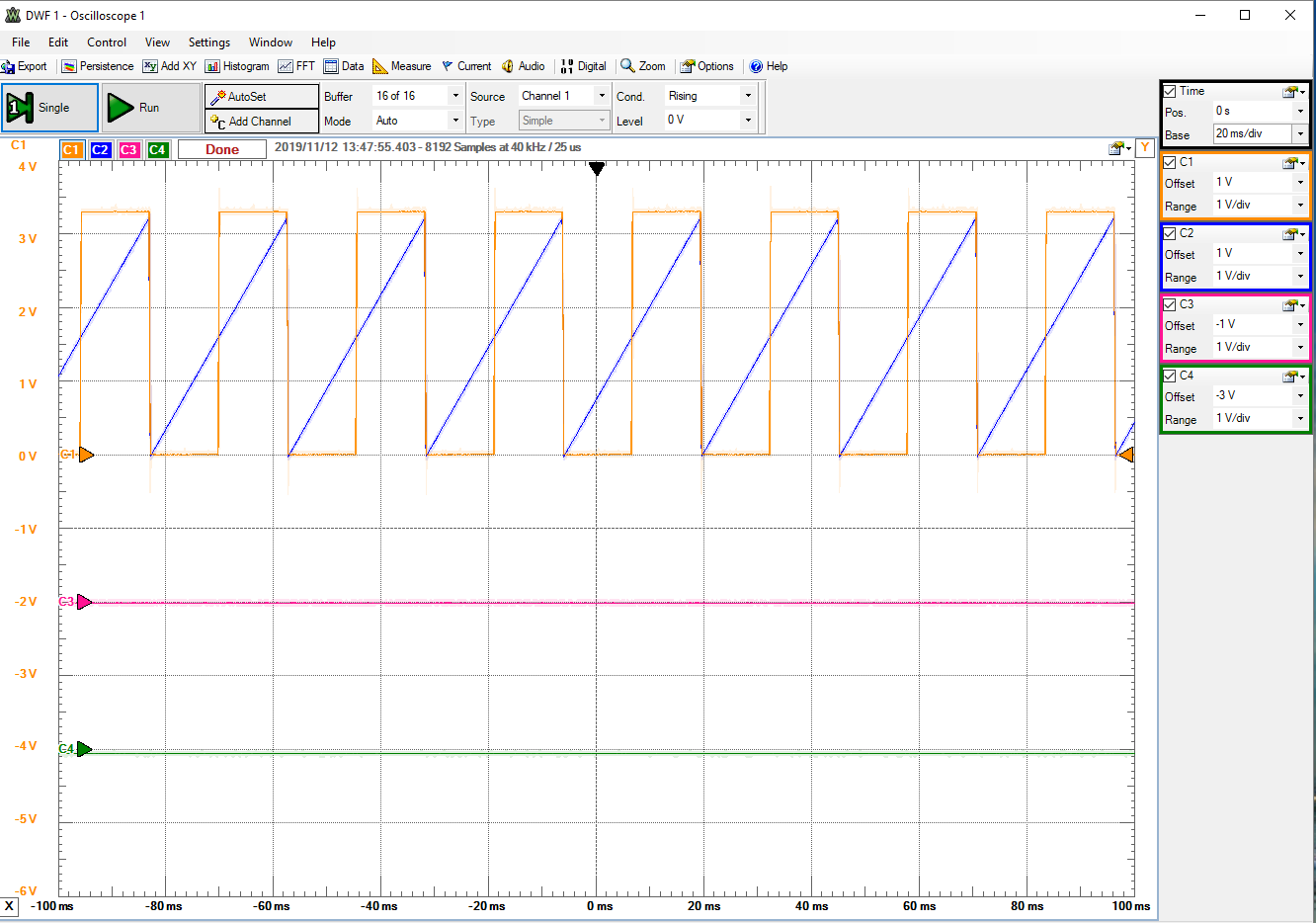figure3:10kHZ frequency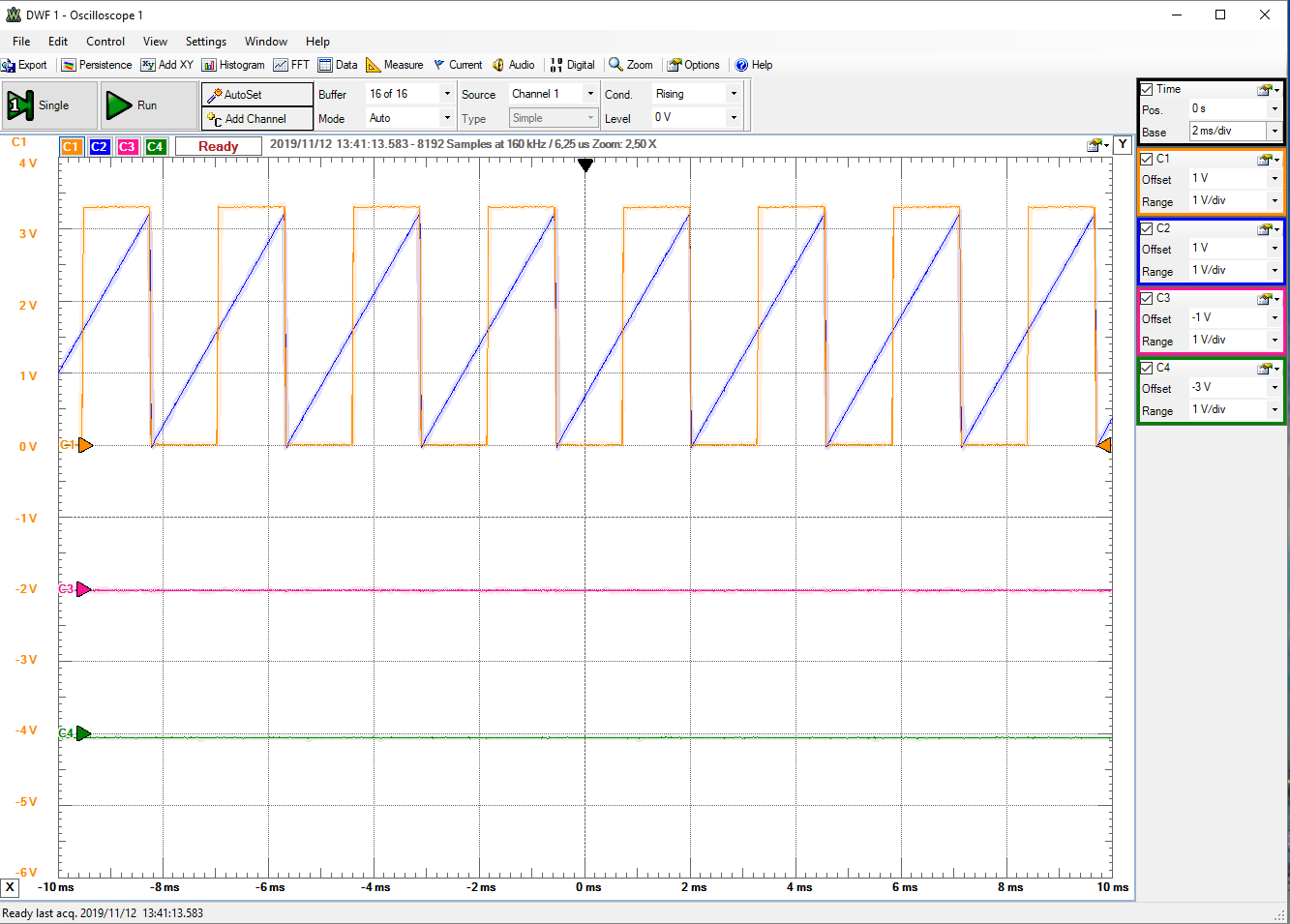figure4: 100kHZ frequency We can see from the graph that the Ramp is getting bad with the increasing frequencies. We got the better results for the frequencies between 2Khz to 10Khz.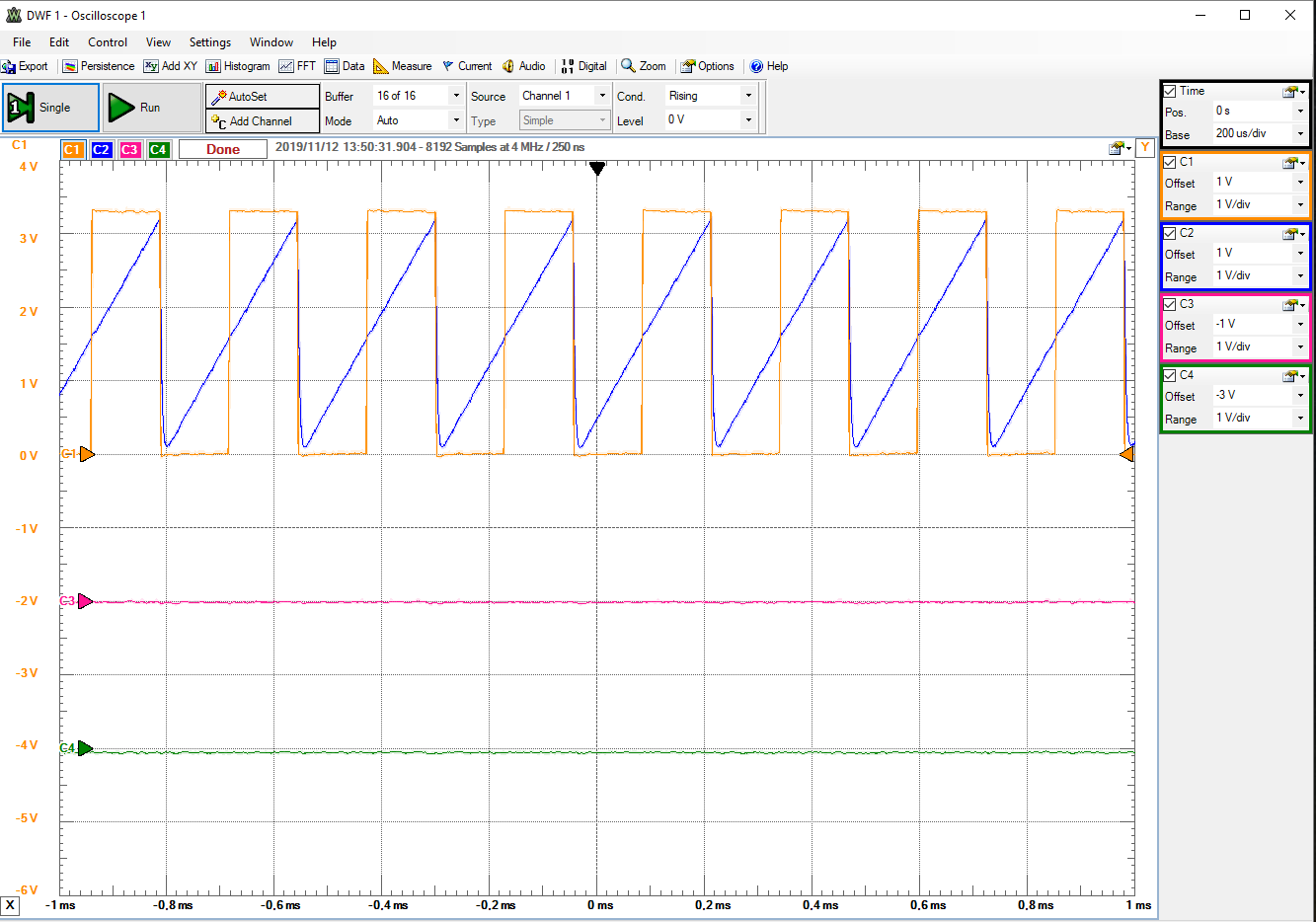figure5:1MHZ frequency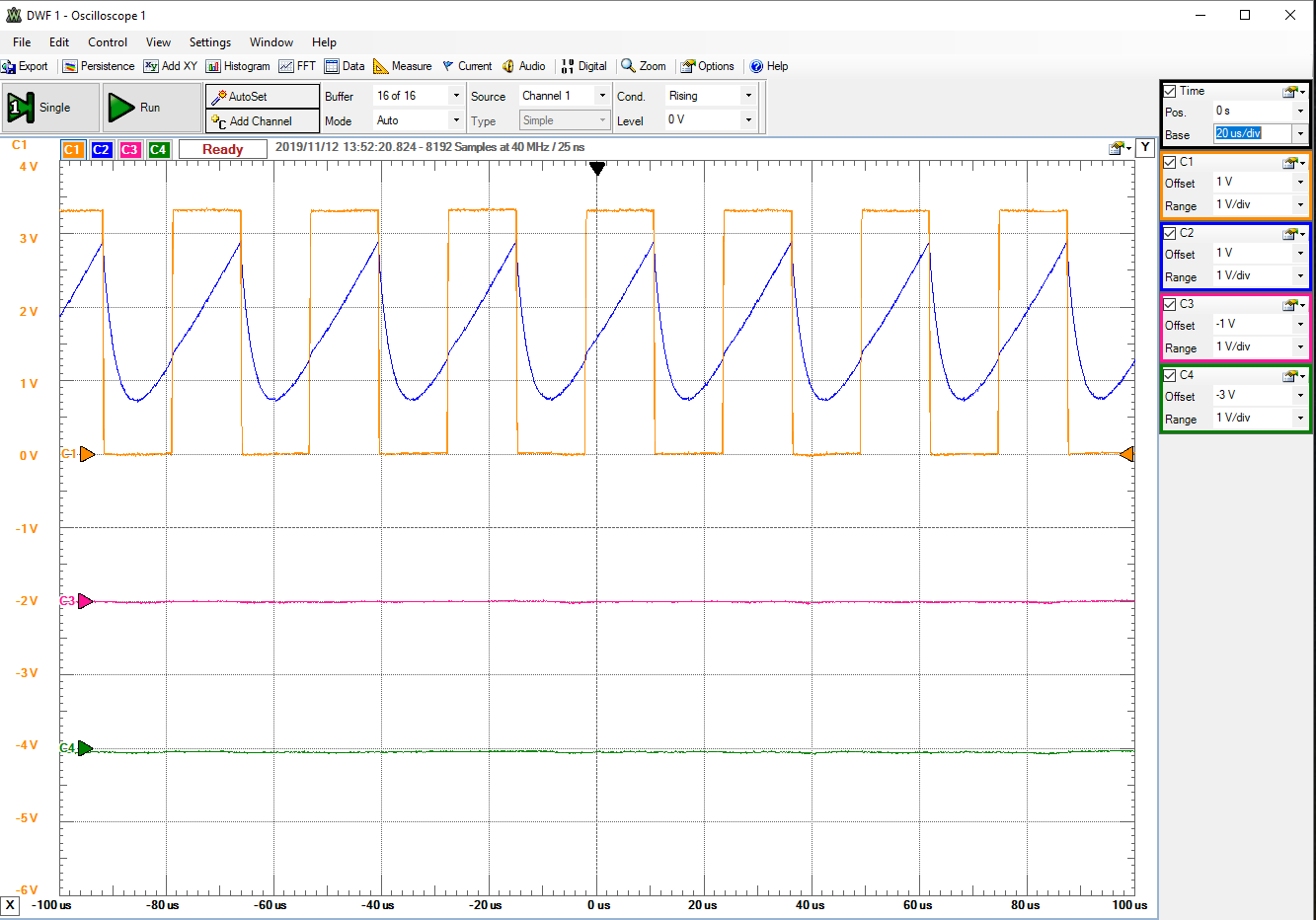figure6:10Mhz frequency

## Settling time of the DAC

 Settling time for the DAC is measured for both full scale and mid scale range. The figure shows the values for rising and falling edge of the clock signal.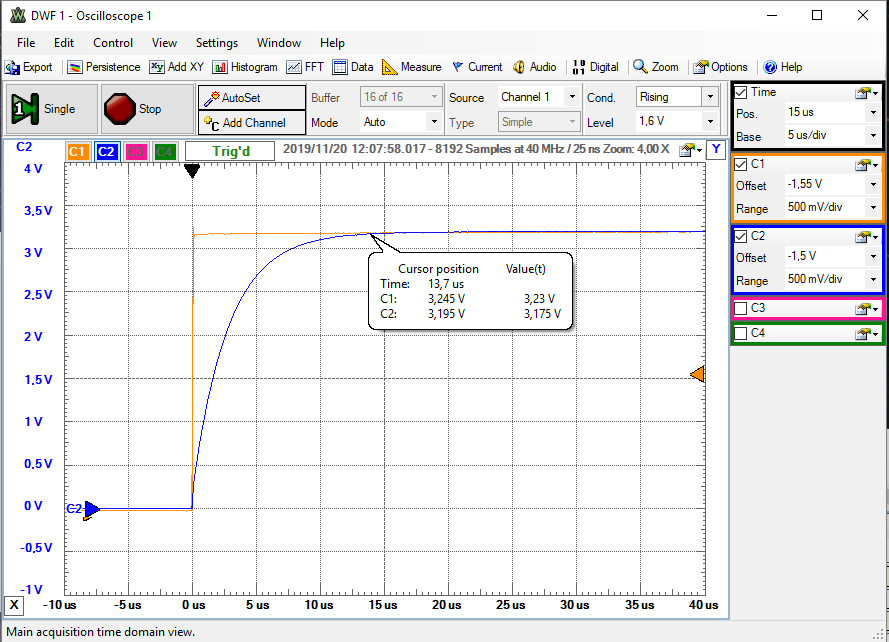figure7: full scale rising time.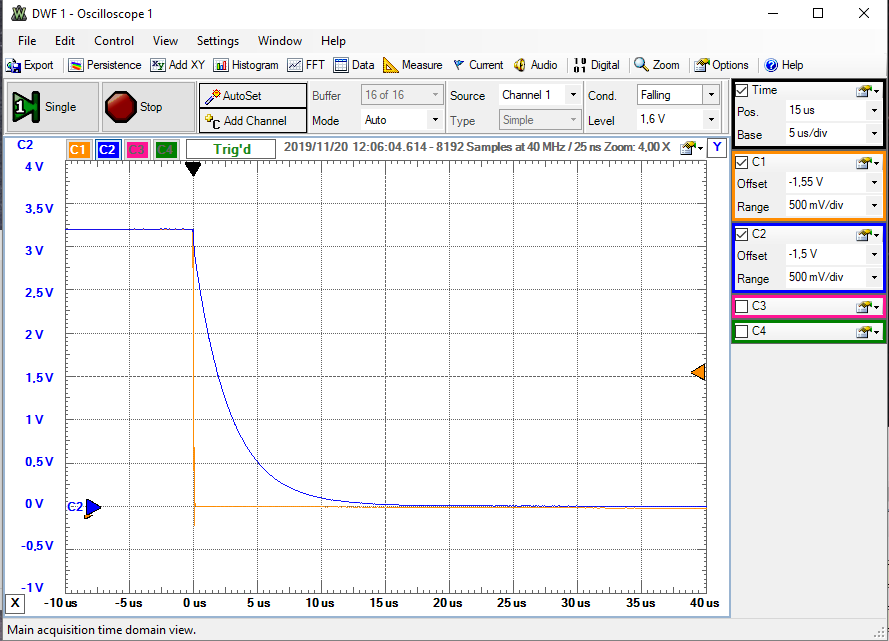figure8:full scale falling time. full scale rising time=13usec full scale falling time=16usec mid scale rising time=1.5usec mid scale falling time=0.049usec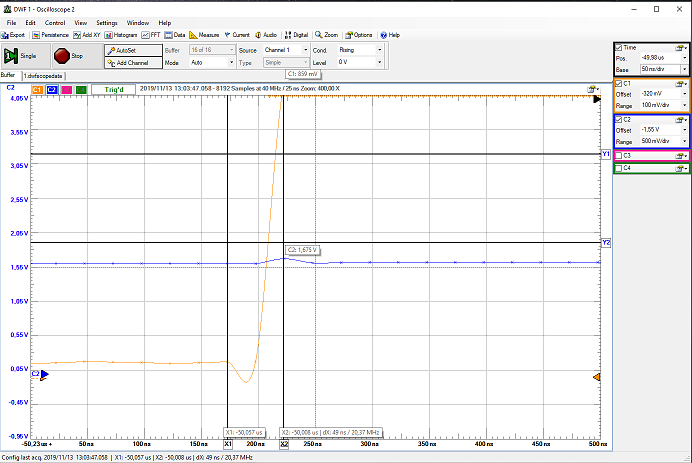figure9:mid scale rising time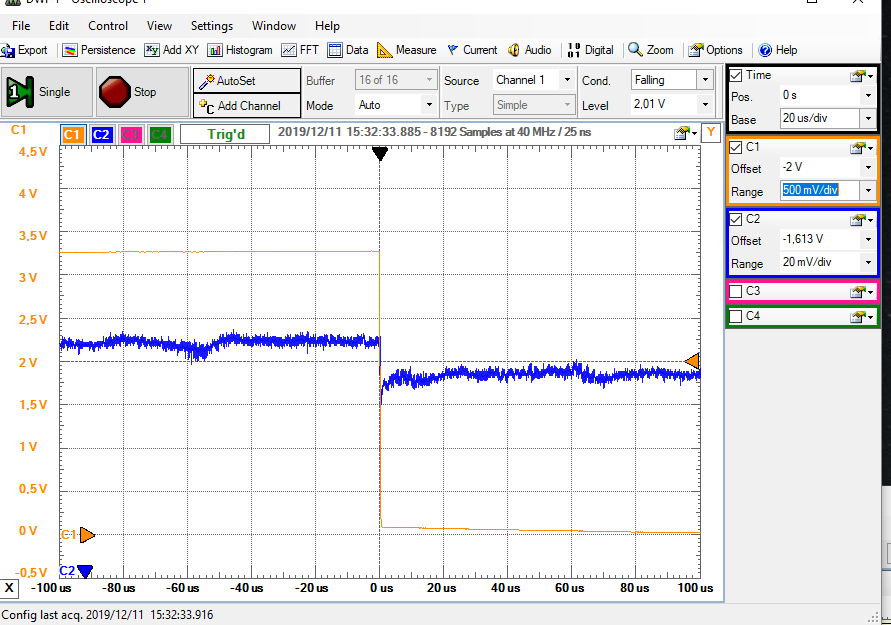figure10:mid scale falling time

## Extraction of INL, DNL

Good extracted ramp data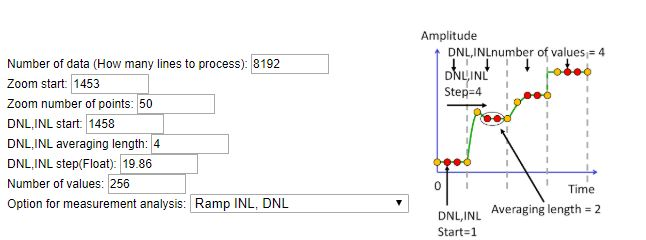figure11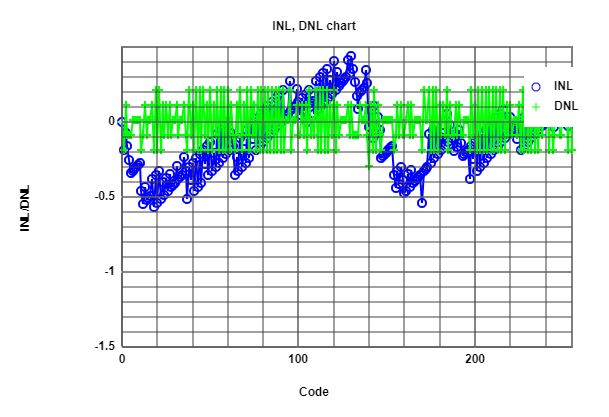figure12: INL,DNL for 2khz frequency
Bad extracted ramp data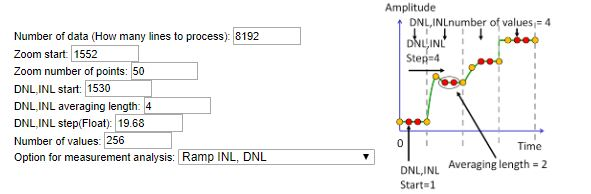figure13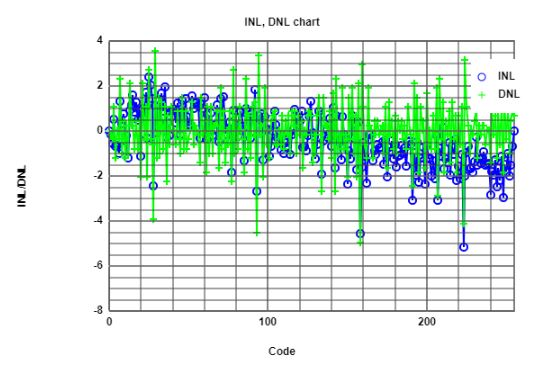figure14:INL,DNL for 200khz frequency

# Challenges and Discussions

• Discussions

• We can see from the above slide that, with the different signal frequencies the values for INL,DNL changes.In case of 2Khz frequency
the range of INL,DNL is between -0.6 to +0.5. And in case of 200khz, the range is between -5 to +4. This value is bad because there will a loss of information

• Challenges
• In simulating the Ramp test the challenges included getting the right value for INL and DNL. We continued working with
much higher frequencies and was not able to get the required accurate values.Challenges also included in getting the specified signal for the analysis.

# Sine measurement of R2R DAC

For the Sine measurement analysis
of DAC we imported the 10bit sine file
with 43 periods from the folder provided.
The results of FFT and SNR is obtained from this.
For both signals FFT shows the highest peak at
around 43HZ ,but the noise level is varying.
Noise level is greater for 50khz than 1khz signal.
From the figure it can also be seen that
ENOB= 7.9435 bits, which is less than 8 bit for 1khz signal.
ENOB= 7.7823 bits, which is less than 8 bit for 50khz signal.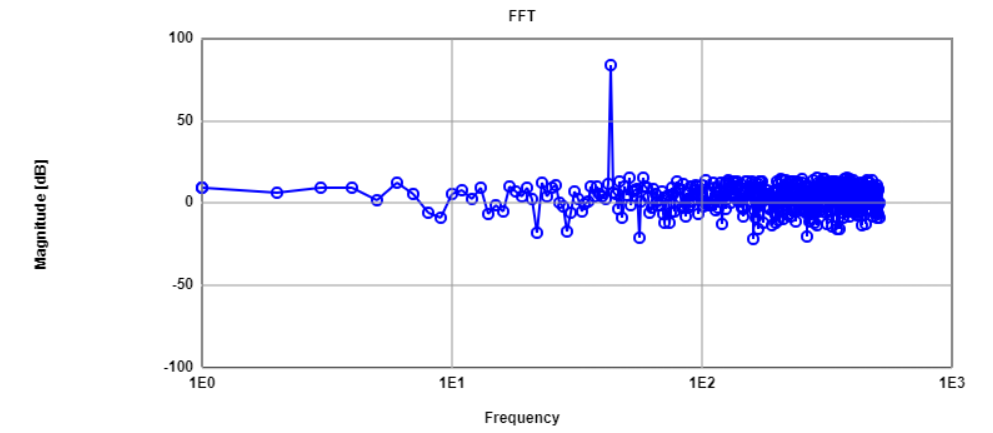figure15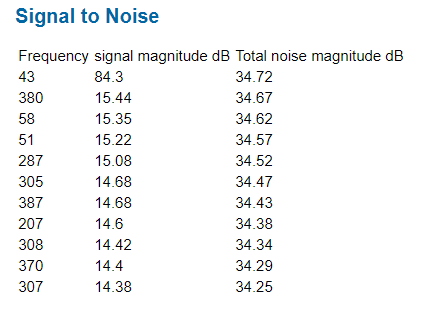figure16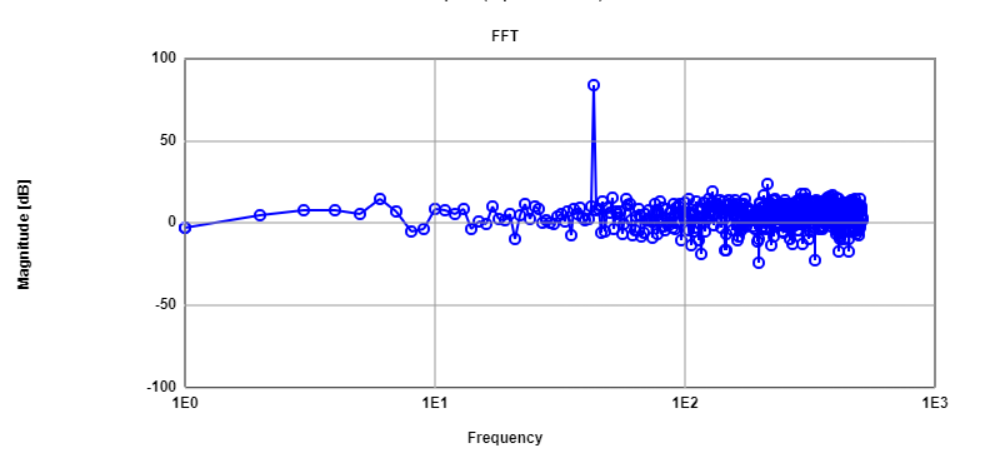figure17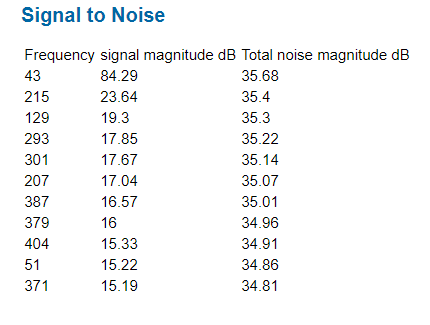figure18

## INL, DNL measurement for different frequencies.

In order to get the comparison for good and bad
sine measurements, we have taken two different
frequencies of 1Khz and 50Khz.
The results in the figure shows that, although
the noise is well under the highest value but as we
increase the frequencies INL,DNL is very much crossing
the limits of +/- 0.5 LSB range.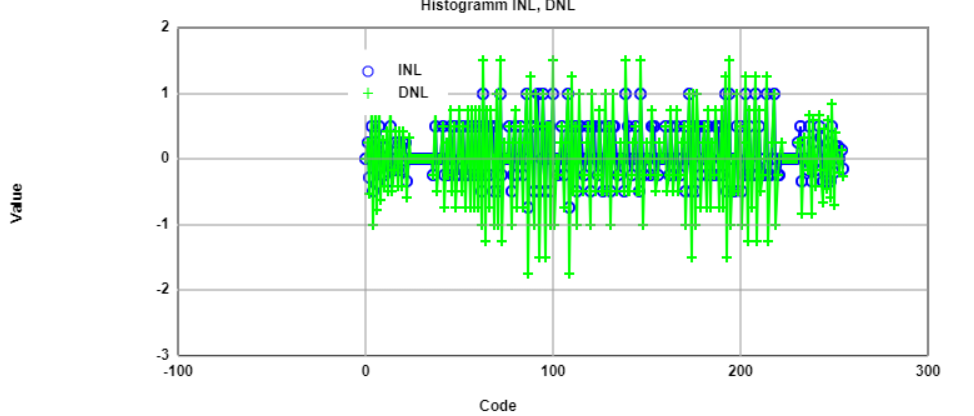figure19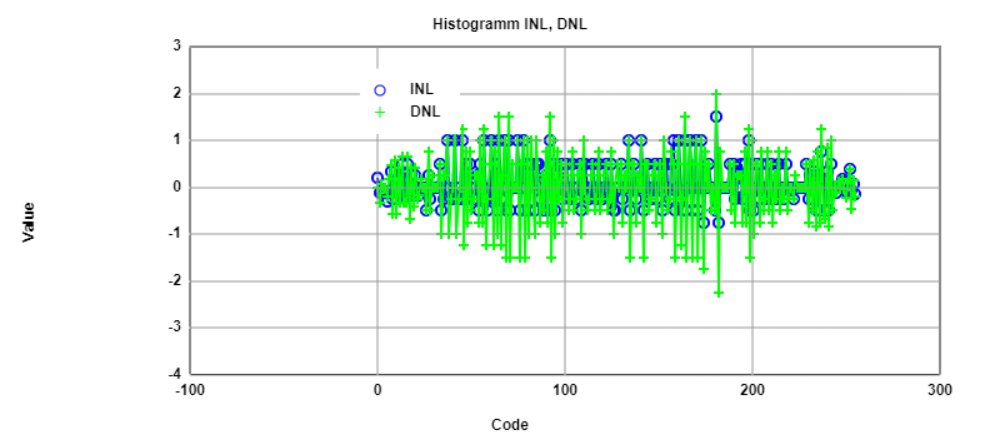figure20

# Digital calibrated R2R DAC

 For the calibration of sine we used the triangular wave and used that values to get the FFT points.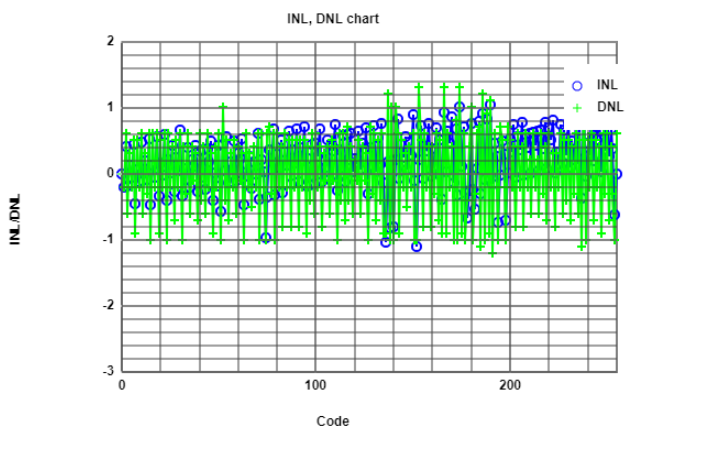figure21 The calibrated data shows the better results for INL,DNL values than the non calibrated data The values of INL, DNL now is in the range of +/- 0.5 LSB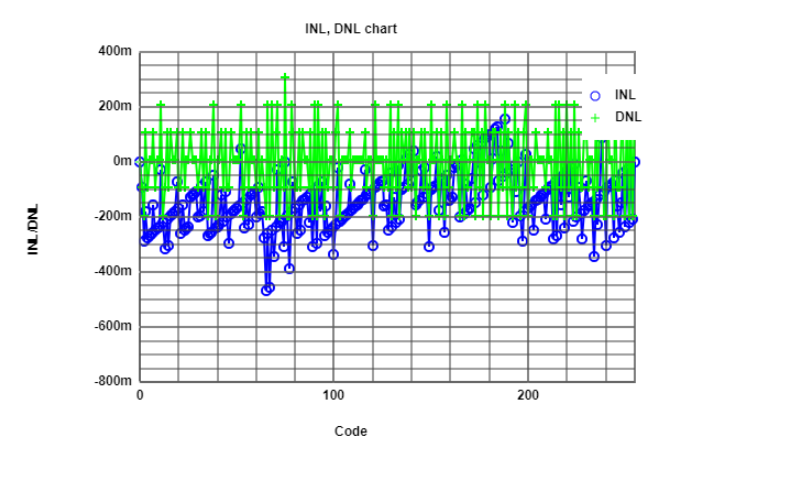figure22

# Summary

• Ramp:
• f measured= 2Khz INL,DNL range= -0.6 to +0.5

• Settling time:
• t_full_scale_up=13usec. t_full_scale_down=16usec. t_mid_scale_up=1.5usec. t_mid_scale_down=0.049usec.

• Sine:
• f_measure=1khz SNR= 84.3-34.72=49.58db SDR=20.86 ENOB=7.9435

• Digital calibrated INL,DNL:
• INL,DNL range= -0.5 to +0.3

# Time Spent and Contribution

The total time spent working on this laboratory was around 25 hours distributed between the lab session
implementing the simulation, analysing the data and working on the report. However, most of the time was spent on
simulating the correct results.We tried to get our best for the results.
Simulation and analysis is done by collaboration of both candidates. However the candidate with matriculation number ***726 carried out the web report until
the sine signal calibration and the candidate with matriculation number ***910 carried out the web report after that part.

# Conclusion

More detailed analysis of the R2R DAC is carried out which helped us clearing with many concepts of analysis.
The calibration methods and the factors affecting that was also learned.